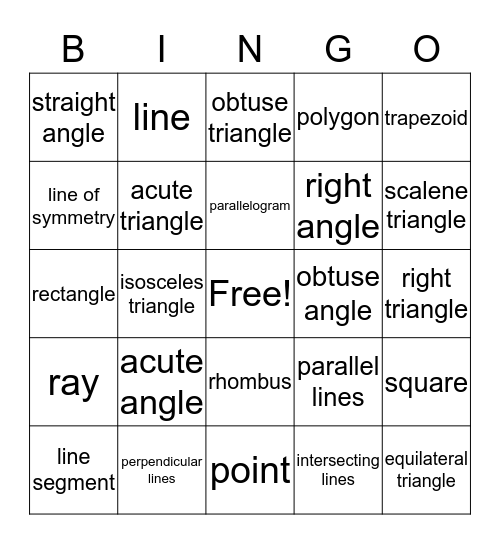Geometry BingoThis bingo card has a free space and 24 words: acute angle, perpendicular lines, parallelogram, line segment, trapezoid, straight angle, line of symmetry, line, obtuse angle, rhombus, right angle, rectangle, parallel lines, square, acute triangle, intersecting lines, right triangle, obtuse triangle, scalene triangle, isosceles triangle, equilateral triangle, ray, polygon and point.

Play Online